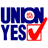DU Home » Latest Threads » Forums & Groups » Main » General Discussion (Forum) » How to calculate the cost...

Mon Feb 27, 2012, 06:41 PM# How to calculate the cost per gallon for gas

According to Ehow. com, this is how it's done:

Break down the per-gallon price of gasoline. According to the U.S. Energy Information Administration, the price of crude oil accounts for about 67 percent of the per-gallon gas price. Another 7 percent is based on the price to refine crude. Distribution and marketing account for 11 percent, and the remaining 15 percent comes from taxes. Keep in mind these percentages can change, particularly because each state has a different gas tax.

Divide the day's crude oil price by 42. One barrel of crude contains 42 gallons. This will tell you the dollar amount per gallon of refined gasoline attributed to crude. For example, if crude oil is \$100 per barrel, then about \$2.38 of the price of a gallon of gas comes from the crude price.

Divide the dollar amount per gallon of refined gas attributed to crude in half. This will give you a dollar amount that accounts for about one-third of the total price per gallon. By multiplying this amount by three, you can get an estimated price per gallon. For example: using \$2.38, divide by two to get \$1.19. Multiply \$1.19 by three to get \$3.57, an average cost per gallon of gasoline.

eHow.com http://www.ehow.com/how_7571180_calculate-price-crude-oil-price.html#ixzz1ncjdxZcM

Today's price per barrel of oil is \$107 so about \$2.54 of the price of gas comes from the crude price. Now the \$2.54 divided by 2=\$1.27 that multiplied by 3=\$3.81 per gallon of gas. So right now it doesn't seem to be any gouging on price. In my neighborhood it's going for \$3.75 a gallon.

0 replies, 29514 views